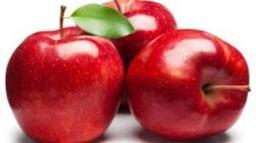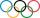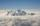# Bitoo and Reena

Bitoo ate 3/5 part of an apple and the remaining part was eaten by his sister Reena. How much part of an apple did Renna eat? Who had the larger share? By how much?

Result

R =  0.4
X = (Correct answer is: B)Y =  0.2

#### Solution:

$B = \dfrac{ 3 }{ 5 } = 0.6 \ \\ R = 1 - B = 1 - 0.6 = \dfrac{ 2 }{ 5 } = 0.4$
$B>R \ \\ \dfrac{ 3 }{ 5 } > \dfrac{ 2 }{ 5 } \ \\ 3>5 \ \\ X = B = 0.6$
$Y = B-R = 0.6-0.4 = \dfrac{ 1 }{ 5 } = 0.2$Our examples were largely sent or created by pupils and students themselves. Therefore, we would be pleased if you could send us any errors you found, spelling mistakes, or rephasing the example. Thank you!

Leave us a comment of this math problem and its solution (i.e. if it is still somewhat unclear...):Be the first to comment!#### Following knowledge from mathematics are needed to solve this word math problem:

Need help calculate sum, simplify or multiply fractions? Try our fraction calculator.

## Next similar math problems:

1. In fractionsAn ant climbs 2/5 of the pole on the first hour and climbs 1/4 of the pole on the next hour. What part of the pole does the ant climb in two hours?
2. ZdeněkZdeněk picked up 15 l of water from a 100-liter full-water barrel. Write a fraction of what part of Zdeněk's water he picked.
3. KingKing had four sons. First inherit 1/2, second 1/4 , third 1/5 of property. What part of the property was left to the last of the brothers?
4. Pizza 4Marcus ate half pizza on monday night. He than ate one third of the remaining pizza on Tuesday. Which of the following expressions show how much pizza marcus ate in total?
5. CupcakesIn a bowl was some cupcakes. Janka ate one third and Danka ate one quarter of cupcakes. a) How many of cookies ate together? b) How many cookies remain in a bowl? Write the results as a decimal number and in notepad also as a fraction.
6. TeacherTeacher Rem bought 360 pieces of cupcakes for the outreach program of their school. 5/9 of the cupcakes were chocolate flavor and 1/4 wete pandan flavor and the rest were a vanilla flavor. How much more pandan flavor cupcakes than vanilla flavor?
7. Cupcakes 2Susi has 25 cupcakes. She gives 4/5. How much does she have left?
8. Passenger boatTwo-fifths of the passengers in the passenger boat were boys. 1/3 of them were girls and the rest were adult. If there were 60 passengers in the boat, how many more boys than adult were there?
9. Fraction and a decimalWrite as a fraction and a decimal. One and two plus three and five hundredths
10. Lengths of the poolMiguel swam 6 lengths of the pool. Mat swam 3 times as far as Miguel. Lionel swam 1/3 as far as Miguel. How many lengths did mat swim?
11. Fraction to decimalWrite the fraction 3/22 as a decimal.
12. Write 2Write 791 thousandths as fraction in expanded form.
13. Mixed2improperWrite the mixed number as an improper fraction. 166 2/3
14. WithdrawalIf I withdrew 2/5 of my total savings and spent 7/10 of that amount. What fraction do I have in left in my savings?
15. RegroupingSubtract mixed number with regrouping: 11 17/20- 6 19/20Pupils doing research which a winter sport do their classmates most popular. They found that 2/5 of classmates would most like to play hockey, skate prefer 2/9 pupils, 3/10 students prefer skiing and 1/15 classmates don't like any winter sport. What proMountain has the elevation 7450 meters and in the morning is the middle portion thereof in the clouds. How many meters of height is in the sky if bellow the clouds is 2,000 meters and above clouds are two fifths of elevation of the mountain?